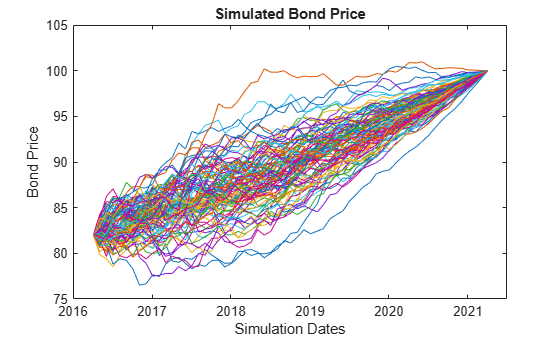HullWhite1F

Create Hull-White one-factor model

Description

The Hull-White one-factor model is specified using the zero curve, alpha, and sigma parameters.

Specifically, the HullWhite1F model is defined using the following equations:

$dr=\left[\theta \left(t\right)-a\left(t\right)r\right]dt+\sigma \left(t\right)dW$

where:

dr is the change in the short-term interest rate over a small interval.

r is the short-term interest rate.

Θ(t) is a function of time determining the average direction in which r moves, chosen such that movements in r are consistent with today's zero coupon yield curve.

α is the mean reversion rate.

dt is a small change in time.

σ is the annual standard deviation of the short rate.

W is the Brownian motion.

Creation

Description

example

HW1F = HullWhite1F(ZeroCurve,Alpha,Sigma) creates a HullWhite1F (HW1F) object using the required arguments to set the Properties.

Properties

expand all

Zero curve, specified as an output from IRDataCurve or a RateSpec that is obtained from intenvset. This is the zero curve used to evolve the path of future interest rates.

Data Types: object | struct

Mean reversion, specified either as a scalar or function handle which takes time as input and returns a scalar mean reversion value.

Data Types: double

Volatility, specified either as a scalar or function handle which takes time as input and returns a scalar mean volatility.

Data Types: double

Object Functions

 simTermStructs Simulate term structures for Hull-White one-factor model

Examples

collapse all

Create a Hull-White one-factor model using an IRDataCurve.

Settle = datenum('15-Dec-2007');
CurveTimes = [1:5 7 10 20]';
ZeroRates = [.01 .018 .024 .029 .033 .034 .035 .034]';

irdc = IRDataCurve('Zero',Settle,CurveDates,ZeroRates);

alpha = .1;
sigma = .01;

HW1F = HullWhite1F(irdc,alpha,sigma)
HW1F =
HullWhite1F with properties:

ZeroCurve: [1x1 IRDataCurve]
Alpha: @(t,V)inAlpha
Sigma: @(t,V)inSigma

Use the simTermStructs method with the HullWhite1F model to simulate term structures.

SimPaths = simTermStructs(HW1F, 10,'nTrials',100);

Create a Hull-White one-factor model using a RateSpec.

Settle = datenum('15-Dec-2007');
CurveTimes = [1:5 7 10 20]';
ZeroRates = [.01 .018 .024 .029 .033 .034 .035 .034]';

RateSpec = intenvset('Rates',ZeroRates,'EndDates',CurveDates,'StartDate',Settle);

alpha = .1;
sigma = .01;

HW1F = HullWhite1F(RateSpec,alpha,sigma)
HW1F =
HullWhite1F with properties:

ZeroCurve: [1x1 IRDataCurve]
Alpha: @(t,V)inAlpha
Sigma: @(t,V)inSigma

Use the simTermStructs method with the HullWhite1F model to simulate term structures.

SimPaths = simTermStructs(HW1F, 10,'nTrials',100);

Define the zero curve data.

Settle = datenum('4-Apr-2016');
ZeroTimes = [3/12 6/12 1 5 7 10 20 30]';
ZeroRates = [0.033 0.034 0.035 0.040 0.042 0.044 0.048 0.0475]';
ZeroDates = datemnth(Settle,ZeroTimes*12);
RateSpec = intenvset('StartDates', Settle,'EndDates', ZeroDates, 'Rates', ZeroRates)
RateSpec = struct with fields:
FinObj: 'RateSpec'
Compounding: 2
Disc: [8x1 double]
Rates: [8x1 double]
EndTimes: [8x1 double]
StartTimes: [8x1 double]
EndDates: [8x1 double]
StartDates: 736424
ValuationDate: 736424
Basis: 0
EndMonthRule: 1

Define the bond parameters.

Maturity = datemnth(Settle,12*5);
CouponRate = 0;

Define the Hull-White parameters.

alpha = .1;
sigma = .01;
HW1F = HullWhite1F(RateSpec,alpha,sigma)
HW1F =
HullWhite1F with properties:

ZeroCurve: [1x1 IRDataCurve]
Alpha: @(t,V)inAlpha
Sigma: @(t,V)inSigma

Define the simulation parameters.

nTrials = 100;
nPeriods = 12*5;
deltaTime = 1/12;
SimZeroCurvePaths = simTermStructs(HW1F, nPeriods,'nTrials',nTrials,'deltaTime',deltaTime);
SimDates = datemnth(Settle,1:nPeriods);

Preallocate and initialize for the simulation.

SimBondPrice = zeros(nPeriods+1,nTrials);
SimBondPrice(1,:,:) = bondbyzero(RateSpec,CouponRate,Settle,Maturity);
SimBondPrice(end,:,:) = 100;

Compute the bond values for each simulation date and path, note that you can vectorize over the trial dimension.

for periodidx=1:nPeriods-1
simRateSpec = intenvset('StartDate',SimDates(periodidx),'EndDates',...
datemnth(SimDates(periodidx),ZeroTimes*12),'Rates',squeeze(SimZeroCurvePaths(periodidx+1,:,:)));
SimBondPrice(periodidx+1,:) = bondbyzero(simRateSpec,CouponRate,SimDates(periodidx),Maturity);
end

plot([Settle SimDates],SimBondPrice)
datetick
ylabel('Bond Price')
xlabel('Simulation Dates')
title('Simulated Bond Price')Define the zero curve data.

Settle = datenum('4-Apr-2016');
ZeroTimes = [3/12 6/12 1 5 7 10 20 30]';
ZeroRates = [-0.01 -0.009 -0.0075 -0.003 -0.002 -0.001 0.002 0.0075]';
ZeroDates = datemnth(Settle,ZeroTimes*12);
RateSpec = intenvset('StartDates', Settle,'EndDates', ZeroDates, 'Rates', ZeroRates)
RateSpec = struct with fields:
FinObj: 'RateSpec'
Compounding: 2
Disc: [8x1 double]
Rates: [8x1 double]
EndTimes: [8x1 double]
StartTimes: [8x1 double]
EndDates: [8x1 double]
StartDates: 736424
ValuationDate: 736424
Basis: 0
EndMonthRule: 1

Define the bond parameters for the five bonds in the portfolio.

Maturity = datemnth(Settle,12*5);  % All bonds have the same maturity
CouponRate = [0.035;0.04;0.02;0.015;0.042];  % Different coupon rates for the bonds
nBonds = length(CouponRate);

Define the Hull-White parameters.

alpha = .1;
sigma = .01;
HW1F = HullWhite1F(RateSpec,alpha,sigma)
HW1F =
HullWhite1F with properties:

ZeroCurve: [1x1 IRDataCurve]
Alpha: @(t,V)inAlpha
Sigma: @(t,V)inSigma

Define the simulation parameters.

nTrials = 1000;
nPeriods = 12*5;
deltaTime = 1/12;
SimZeroCurvePaths = simTermStructs(HW1F, nPeriods,'nTrials',nTrials,'deltaTime',deltaTime);
SimDates = datemnth(Settle,1:nPeriods);

Preallocate and initialize for the simulation.

SimBondPrice = zeros(nPeriods+1,nBonds,nTrials);
SimBondPrice(1,:,:) = repmat(bondbyzero(RateSpec,CouponRate,Settle,Maturity)',[1 1 nTrials]);
SimBondPrice(end,:,:) = 100;

[BondCF,BondCFDates,~,CFlowFlags] = cfamounts(CouponRate,Settle,Maturity);
BondCF(CFlowFlags == 4) = BondCF(CFlowFlags == 4) - 100;
SimBondCF = zeros(nPeriods+1,nBonds,nTrials);

Compute bond values for each simulation date and path. Note that you can vectorize over the trial dimension.

for periodidx=1:nPeriods
if periodidx < nPeriods
simRateSpec = intenvset('StartDate',SimDates(periodidx),'EndDates',...
datemnth(SimDates(periodidx),ZeroTimes*12),'Rates',squeeze(SimZeroCurvePaths(periodidx+1,:,:)));
SimBondPrice(periodidx+1,:,:) = bondbyzero(simRateSpec,CouponRate,SimDates(periodidx),Maturity);
end

simidx = SimDates(periodidx) == BondCFDates;
SimCF = zeros(1,nBonds);
SimCF(any(simidx,2)) = BondCF(simidx);
ReinvestRate = 1 + SimZeroCurvePaths(periodidx+1,1,:);
SimBondCF(periodidx+1,:,:) = bsxfun(@times,bsxfun(@plus,SimBondCF(periodidx,:,:),SimCF),ReinvestRate);
end

Compute the total return series.

TotalCF = SimBondPrice + SimBondCF;

Assume the bond portfolio is equally weighted and plot the simulated bond portfolio returns.

TotalCF = squeeze(sum(TotalCF,2));

TotRetSeries = bsxfun(@rdivide,TotalCF(2:end,:),TotalCF(1,:)) - 1;
plot(SimDates,TotRetSeries)
datetick
ylabel('Bond Portfolio Returns')
xlabel('Simulation Dates')
title('Simulated Bond Portfolio Returns')